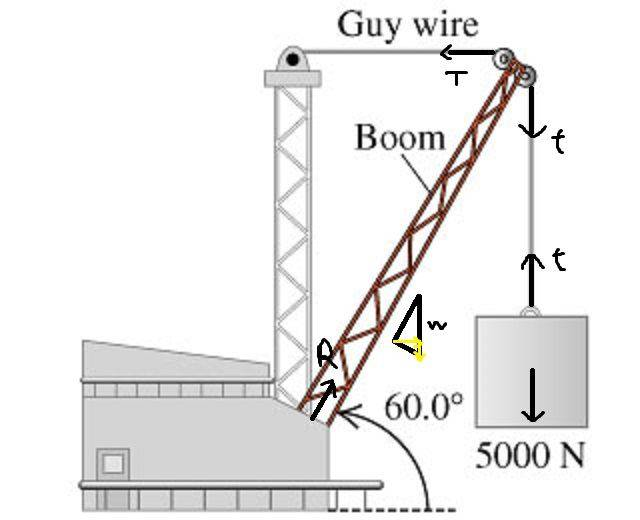# Static equilibrium problem: Finding tension

• archaic
In summary, when considering the diagonal component of a boom's weight as a reaction, it can be decomposed into horizontal and vertical components, and the vertical component can be used to calculate the weight of the boom. However, in order to find the correct solution, it is important to also decompose the weight counterpart of the reaction and use the torque method to solve the problem. It is recommended to double check all calculations and follow the guidelines given by your teachers for significant figures.

#### archaic

Homework Statement
The boom in the figure below weighs 3100 N and is attached to a frictionless pivot at its lower end. It is not uniform; the distance of its center of gravity from the pivot is 35.0 % of its length.
Relevant Equations
$$\sum\vec F=\vec0\\\sim\vec\tau=\vec0$$The diagonal component of the boom's weight is ##R=w\cos\frac{\pi}{6}=\frac{\sqrt 3}{2}w##, and, considering ##R## as a "reaction", we have ##R_x=R\cos\frac{\pi}{3}=\frac{\sqrt 3}{4}w## and ##R_y=R\sin\frac{\pi}{3}=\frac 34w##.
I will also have ##w_x=w\sin\frac{\pi}{6}\cos\frac{\pi}{6}=\frac{\sqrt 3}{4}w## and ##w_y=w\sin\frac{\pi}{6}\sin\frac{\pi}{6}=\frac 14w##.
$$\sum F_y=5000+w_y-R_y=5000+\frac 14w-\frac 34w=0\\w=10000\,N \\\sum F_x=T-R_x-w_x=T-\frac{\sqrt3}{4}w-\frac{\sqrt 3}{4}w=0\\T=5000\sqrt3\,N$$

Last edited:
I messed up, probably because of ##R##. Let me consider the torques about the axis through the point of application of ##R## (in the image).
$$\sum\tau=0\Leftrightarrow lT\sin\frac{2\pi}{3}-5000l\sin\frac{5\pi}{6}-0.35\times5000l\sin\frac{5\pi}{6}=0\\T\sin\frac{2\pi}{3}-1.35\times5000\sin\frac{5\pi}{6}=0\\T=3375\,N$$

At your picture the reaction R is directed along the boom Why? A priori you do not know its direction

archaic said:
I messed up, probably because of ##R##. Let me consider the torques about the axis through the point of application of ##R## (in the image).
$$\sum\tau=0\Leftrightarrow lT\sin\frac{2\pi}{3}-5000l\sin\frac{5\pi}{6}-0.35\times5000l\sin\frac{5\pi}{6}=0\\T\sin\frac{2\pi}{3}-1.35\times5000\sin\frac{5\pi}{6}=0\\T=3375\,N$$
You seem to have used 5000N for both weights.

Wrt @wrobel 's point about the direction of R, consider moments about the top of the boom. If R acts along the boom then the weight of the boom is the only force with a torque about that axis.

•archaic
wrobel said:
At your picture the reaction R is directed along the boom Why? A priori you do not know its direction
I didn't really consider reaction; I tried to come up with a force in the direction of ##T## so I decomposed the boom's weight in the way shown, but that makes a diagonal force that needs to be cancelled, hence me supposing ##R##.
The wrong step I did in summing up the forces was decomposing ##R## without decomposing its weight counterpart.
I need a vertical force to find the boom's weight, but I still don't see where it is.
...

haruspex said:
You seem to have used 5000N for both weights.

Wrt @wrobel 's point about the direction of R, consider moments about the top of the boom. If R acts along the boom then the weight of the boom is the only force with a torque about that axis.
Hm, right. I can also find ##R_\perp=-\frac{13}{40l}w##. Don't see how that helps me, though.

archaic said:
I need a vertical force to find the boom's weight, but I still don't see where it is.
...
The weight of the boom is given, 3100 N. And the force from the pivot does not act along the length of the boom: it has both vertical and horizontal components. No use to count with it. Solve the problem with the torque method.

ehild said:
The weight of the boom is given, 3100 N. And the force from the pivot does not act along the length of the boom: it has both vertical and horizontal components. No use to count with it. Solve the problem with the torque method.
I forgot about it being given... Thank you.

$$\sum\tau=0\Leftrightarrow lT\sin\frac{\pi}{3}-5000l\sin\frac{\pi}{6}-0.35\times3100l\sin\frac{\pi}{6}=0\\T\sin\frac{\pi}{3}-6085\sin\frac{\pi}{6}=0\\T=\frac{6085}{\sqrt 3}\approx351.\times10^1\,N$$
I think this is good. Afraid of checking on the platform, though. Anyone can confirm?

archaic said:
I forgot about it being given... Thank you.

$$\sum\tau=0\Leftrightarrow lT\sin\frac{\pi}{3}-5000l\sin\frac{\pi}{6}-0.35\times3100l\sin\frac{\pi}{6}=0\\T\sin\frac{\pi}{3}-6085\sin\frac{\pi}{6}=0\\T=\frac{6085}{\sqrt 3}\approx351.\times10^1\,N$$
Correct, but why do you give it in 10 Newtons, instead of Newtons, , with two significant digits?

ehild said:
Correct, but why do you give it in 10 Newtons, instead of Newtons, , with two significant digits?
Thank you. It is in three significant figures, since I'm taking ##35.0\%## as having that number of SFs.

archaic said:
Thank you. It is in three significant figures, since I'm taking ##35.0\%## as having that number of SFs.
You are right! But even then, you can write it out as 3510 N , or 3.51x103 N

ehild said:
You are right! But even then, you can write it out as 3510 N , or 3.51x103 N
Oh, the way I learned it from chemistry is that abc.x10n counts also as three significant figures.

archaic said:
Oh, the way I learned it from chemistry is that abc.x10n counts also as three significant figures.
It is correct, only 101 looks strange. Do as it is required by your teachers

•Delta2 and archaic
archaic said:
I didn't really consider reaction;
I see but this is not the reason to write wrong things

archaic said:
Hm, right. I can also find ##R_\perp=-\frac{13}{40l}w##. Don't see how that helps me, though.
It doesn’t.. just illustrating that the reaction cannot be along the boom.

•archaic
haruspex said:
It doesn’t.. just illustrating that the reaction cannot be along the boom.
Yes, I understood your point; "I can also..". Thank you!